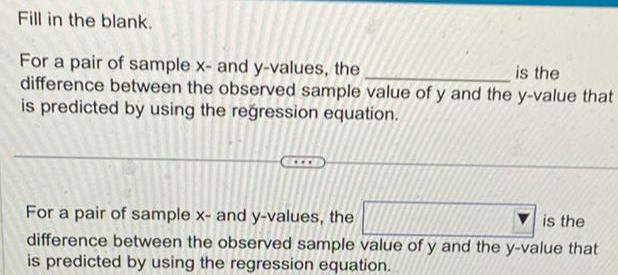Question:

# Fill in the blank is the For a pair of sample x and y values

Last updated: 11/20/2023Fill in the blank is the For a pair of sample x and y values the difference between the observed sample value of y and the y value that is predicted by using the regression equation For a pair of sample x and y values the is the difference between the observed sample value of y and the y value that of ya is predicted by using the regression equation